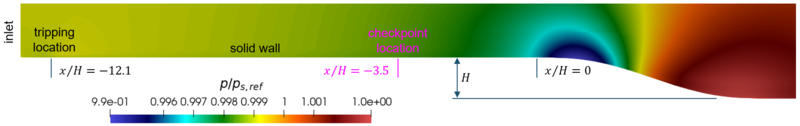# Introduction

The HiFi-TURB-DLR rounded step test case is a case in which a turbulent boundary layer flows over a flat plate before reaching a planar rounded backward step. The particular geometry generates an adverse pressure gradient which accelerates the boundary layer upstream of the step before inducing an incipient separation above the smooth step, a flow physics that is well known to be not correctly predicted by state-of-the-art Reynolds-Averaged Navier-Stokes (RANS) models.

# Review of previous studies

The design of the test case was inspired by the experimental work on the flow around an axisymmetric test body of Disotell et al. and Disotell et al. (2017). Disotell et al. focused their study of the possible separation and reattachment in the rear part of the body. The original test case is here revised as a 2D planar flow problem without any tunnel walls but with a far-field condition opposite to the solid wall. The reasons for using a planar geometry are thoroughly discussed in UFR 3-36 Test Case.

# Description of the test case

## Geometry and flow parameters

The test case is designed as a numerical experiment with the aim of comparing RANS results to DNS data. The flow problem was defined by DLR with the aid of RANS calculations to get a condition with incipient separation. Other cases with moderate and full separation were also designed by DLR, see Alaya et al. (2021), but for the present uDNS only the incipient condition for the Reynolds number ${Re_{H}=78,490}$and the Mach number $Ma=0.13455$was considered, where $H$is the step height. The geometry of the curved step is shown in Fig. 2 and its definition is reported in UFR 3-36 Test Case.

Figure 2: Set-up for the DNS simulation and pressure distribution## Computation domain and boundary conditions

Although for DNS the inflow boundary conditions are different than RANS, to allow a valid comparison they must guarantee the same boundary layer properties at a given point, hereinafter referred to as checkpoint, upstream of the rounded step, i.e., ${x_{ckp}/H=-3.5}$. At this position ${x_{ckp}}$, the properties to be matched are the Reynolds number based on the momentum thickness ${Re_{\theta }=1,780}$, the Reynolds number based on the friction velocity ${Re_{\tau }=700}$and the boundary layer thickness $\delta _{99}/H=0.241$. As a technique to promote the laminar-turbulent transition of the boundary layer and reduce the upstream length of the domain, a local tripping term inspired by the work of Housseini et al. (2016) and Schlatter and Örlü (2012) is used. To define the mesh density and the computational domain size that ensure the target boundary layer integral parameters at ${x_{chp}}$, a precursory computational campaign for the turbulent flow over a flat plate was performed. According to the outcomes of this campaign, the inlet boundary is located at ${x/H=-12.7}$, where the Blasius laminar velocity profile computed at ${Re_{x}=650,000}$, the uniform static pressure ${P_{s,ref}}$and the uniform total temperature ${T_{t,inflow}}$are imposed, see UFR 3-36: Table 2 for dimensional values of each quantity. At location ${x/H=-12.1}$, within the laminar boundary layer region, the tripping source term is activated to promote the transition to turbulence, see Fig. 2. At the outlet boundary, placed at $x/H=24.0$, the static pressure ${P_{s,ref}}$is imposed with an exit-pressure outflow boundary condition. To mitigate spurious perturbations possibly originating at the outlet boundary, the mesh is coarsened in the streamwise direction for ${x/H>13.82}$. The upper boundary is a permeable far-field Riemann boundary condition located $180.0H$from the no-slip adiabatic wall downstream the smooth step and computed via the exact Riemann solver. Finally, side planes are considered as periodic with a distance from each other of ${\Delta z=3H}$.